Power Release
When Energy is specified, the Power Release method is used to calculate the Turbine Release required. If the Energy request can not be met, the user is notified. There is one method per Power method.
The Power Release category is available when any of the Power methods is selected except None, Peak Power, Peak and Base, or Peak Power Equation.None
This is the default method in the Power Release category. No calculations are performed in this method.
If this method is selected for the Power Release category, a RiverWare error will be posted and the simulation run will be terminated. A viable power release method must be selected when the Power Release category is visible.
There are no slots specific to this method.Plant Power Coefficient Release
The Plant Power Coefficient Release method calculates Turbine Release using the entire plant characteristics when Energy is specified. The Plant Power Coefficient Release method is only available if the Plant Power Coefficient method is selected in the Power category. Energy must be input for this method to execute. If Energy is flagged as either MAX CAPACITY or BEST EFFICIENCY, it is considered input. If Energy is flagged as MAX CAPACITY, Turbine Release is set to meet the Energy request at the maximum flow rate. If Energy is flagged as BEST EFFICIENCY, Turbine Release is set to meet the Energy request at the most efficient flow rate. If Energy is neither flagged as MAX CAPACITY nor flagged as BEST EFFICIENCY, the Turbine Release is calculated from the Energy request and a Power Coefficient. The Power Coefficient may be input by the user or calculated by RiverWare from interpolation of the Best and Max Power Coefficient tables.
If Energy is flagged UNIT VALUES (U), an error is issued. This flag is only available with the Unit Power Table Release method; see Unit Power Table Release.
There are no slots specific to this method.
Method Details
The first step in the Plant Power Coefficient Release algorithm is to set the Power Plant Cap Fraction to 1.0 if it is not already known.
If the Energy slot is flagged as MAX CAPACITY, the following steps are taken.
1. Qtemp, a local variable, is calculated from interpolation of the Max Turbine Q table using the Operating Head.
2. Turbine Release is calculated with the following equation: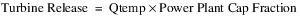3. PCmax, a local variable, is determined from interpolation of the Max Power Coefficient table using the Operating Head. The Power Coefficient is set as PCmax if it is not input.
4. Power and Energy are then calculated using the following equations: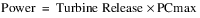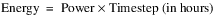5. If the Plant Power Limit is exceeded, Power is reduced to the Plant Power Limit and the Energy is recalculated. A new Power Coefficient and Turbine Release are then calculated based on the Plant Power Limit.
If Energy is flagged as BEST EFFICIENCY, the following steps are taken.
1. Qtemp is calculated from interpolation of the Best Turbine Q table using the Operating Head.
2. Turbine Release is computed using the following equation:3. PCbest, a local variable, is determined from interpolation of the Best Power Coefficient table using the Operating Head. The Power Coefficient is set as PC best if it is not input.
4. Power and Energy are then calculated using the following equations: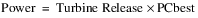5. If the Plant Power Limit is exceeded, Power is reduced to the Plant Power Limit, the Energy is recalculated, and the Turbine Release is recalculated as Plant Power Limit / PCbest.
If Energy is not flagged as either MAX CAPACITY or BEST EFFICIENCY and the Power Coefficient is input, the following steps are taken.
1. If the Power Coefficient is less than 0.00000001, a RiverWare error is posted and the simulation run is terminated.
2. Power is calculated using the following equation: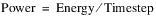where the Timestep is in hours.
3. Qout, a local variable, is calculated with the following equation:4. Qtemp, a local variable, is determined by the interpolation of the Max Turbine Q table using the Operating Head.
5. Qmax, a local variable, is computed using the following equation: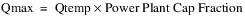6. If Qout is greater than Qmax, the largest discharge value in the Max Turbine Q table is found. If this value is greater than or equal to Qout, Turbine Release is set equal to Qmax. If the value is less than Qout, a RiverWare error is posted and the simulation run is terminated.
7. If Qout is less than or equal to Qmax, Turbine Release is set equal to Qout.
If Energy is not flagged as either MAX CAPACITY or BEST EFFICIENCY and the Power Coefficient is not given, the following steps are taken.
1. Power is calculated using the following equation:where the timestep is in hours.
2. The best and max power coefficients are interpolated using the Operating Head and the Best Power Coefficient and the Max Power Coefficient tables, respectively.
3. QbestTemp and QmaxTemp (local variables) are then determined using the Operating Head to interpolate values from the Best Turbine Q and Max Turbine Q tables, respectively.
4. Qbest, a local variable, is computed using the following equation: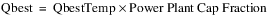5. Qmax, a local variable, is calculated using the following equation: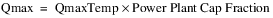6. If Power divided by the best power coefficient is less than or equal to Qbest, Turbine Release is set equal to Power divided by the best power coefficient.
7. If Power divided by the max power coefficient is greater than Qmax, Turbine Release is set equal to the max turbine flow.
8. If neither 3) nor 4) is true, an interpolated value (pcoeffINTERP) is found between the best and max power coefficients based on how close Power is to both the product of Qbest and the best power coefficient, and the product of Qmax and the max power coefficient. The following pair of equations is used to quantitatively determine the pcoeffINTERP value: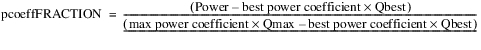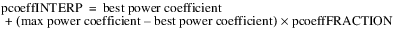9. The Turbine Release is then calculated with the following equation: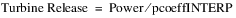Plant Efficiency Curve Release
The Plant Efficiency Curve Release method calculates Turbine Release using the entire plant characteristics when Energy is specified. The Plant Efficiency Curve Release method is only available if the Plant Efficiency Curve method is selected in the Power category. Energy must be input or set by a rule for this method to execute. If Energy is flagged as either MAX CAPACITY or BEST EFFICIENCY, it is considered input. If Energy is flagged as MAX CAPACITY, Turbine Release is set to meet the Energy request at the maximum flow rate. If Energy is flagged as BEST EFFICIENCY, Turbine Release is set to meet the Energy request at the most efficient flow rate. If Energy is neither flagged as MAX CAPACITY nor flagged as BEST EFFICIENCY, the Turbine Release is calculated from the Energy request.
If Energy is flagged UNIT VALUES (U), an error is issued. This flag is only available with the Unit Power Table Release method; see Unit Power Table Release.
There are no slots specific to this method.
Method Details
The first step in the Plant Efficiency Curve Release algorithm is to set the Power Plant Cap Fraction to 1.0 if it is not already known.
If the Energy slot is flagged as MAX CAPACITY, the following steps are taken.
1. Qtemp, a local variable, is calculated as the maximum release using the Operating Head and the Plant Power Table.
2. Turbine Release is calculated with the following equation:3. Power is determined directly from the Plant Power Curve.
4. Energy is calculated as follows: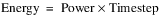5. The Power Coefficient is calculated as follows: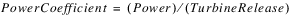6. If the Plant Power Limit is exceeded, Power is reduced to the Plant Power Limit and the Energy is recalculated. A new Power Coefficient and Turbine Release are then calculated based on the Plant Power Limit.
If Energy is flagged as BEST EFFICIENCY, the following steps are taken.
1. Qtemp is computed as the most efficient release given the Operating Head and the Plant Power Table.
2. Turbine Release is computed using the following equation:3. Power is determined directly from the Plant Power Curve.
4. Energy is calculated as follows:5. The Power Coefficient is calculated as follows: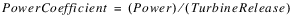6. If the Plant Power Limit is exceeded, Power is reduced to the Plant Power Limit and the Energy is recalculated. A new Power Coefficient and Turbine Release are then calculated based on the Plant Power Limit.
If Energy is not flagged as either MAX CAPACITY or BEST EFFICIENCY and the Power Coefficient is input, the following steps are taken.
1. If the Power Coefficient is less than 0.00000001, a RiverWare error is posted and the simulation run is terminated.
2. Power is calculated using the following equation:3. Turbine Release is calculated as follows:If Energy is not flagged as either MAX CAPACITY or BEST EFFICIENCY and the Power Coefficient is not input, the following steps are taken.
1. Power is calculated using the following equation:2. The max Turbine Release and Power production are found for the current operating conditions.
3. If input Power is greater than the max Power for current operating conditions, and INPUT_ENERGY _ADJUST method is chosen, Turbine Release is set equal to the max Turbine Release from 2, and Power is set equal to Power from 2. The Power Coefficient is then computed as Power divided by Turbine Release.
4. Otherwise, Turbine Release is found using the Plant Power Table and the Power Coefficient is set as Power divided by Turbine Release.
5. If the Plant Power Limit is exceeded, an error is posted.
Note:  If the Power Plant Cap Fraction is input by the user, it is necessary for the Plant Power Table to basically be scaled back to account for the operating points when the turbines are operating at less than 100%. To do this, when Turbine Release is known and Power is to be found using the Plant Power Curve, Turbine Release is divided by the Power Plant Cap Fraction. This point is then found in the Plant Power Curve for the current operating head and the Power is found using 3‑D interpolation. Finally the Power is multiplied by the Power Plant Cap Fraction to get the actual Power produced for the current timestep.
Note:  If Power is known, and Turbine release is to be found in the table. Power is multiplied by the Power Plant Cap Fraction and then this point is found in the Plant Power Curve to solve for Turbine Release. Turbine Release is then divided by the Power Plant Cap Fraction to get the actual Turbine Release for the current timestep.Plant Power Equation Release
The Plant Power Equation Release method calculates Turbine Release using the water power equation when Energy is specified. The Plant Power Equation Release method is only available if the Plant Power Equation method is selected in the Power category. Energy must be input for this method to execute. If Energy is flagged as either MAX CAPACITY or BEST EFFICIENCY, it is considered input. If Energy is flagged as MAX CAPACITY, Turbine Release is set to meet the Energy request at the maximum possible turbine release. If Energy is flagged as BEST EFFICIENCY, the run terminates because BEST EFFICIENCY is not supported in this method.
If Energy is flagged UNIT VALUES (U), an error is issued. This flag is only available with the Unit Power Table Release method; see Unit Power Table Release.
There are no slots specific to this method.
Method Details
This method first checks to see if Turbine Release is user input or set by a rule. If it is, the run terminates because both Energy and Turbine Release cannot be input.
If the Energy slot is flagged as MAX CAPACITY, the following steps are taken.
1. Set Turbine Release to be the maximum turbine release calculated by interpolating the Net Head on the Net Head Vs Max Turbine Release table.
2. Once efficiency, Plant Cap Fraction, Net Head, and Turbine Release are all known, Power is solved for using the Power Equation. The unit compatibility factor comes from balancing units and is 102.01697767 in internal RiverWare units.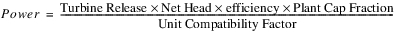If the computed Power is greater than the Plant Power Limit, the Power is reset to the Plant Power Limit. In this case, Turbine Release is recomputed using the previous equation rearranged.
3. Lastly, Energy is computed as Power multiplied by the length of the timestep.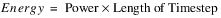If the Energy slot is not flagged MAX CAPACITY, the following steps are taken.
When the Energy value is known (rather than flagged Max Capacity), the Plant Power Equation Release method uses Energy to solve for Power and Turbine Release. Power is simply Energy divided by the length of the timestep: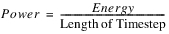Using Power, the Net Head and Turbine Release are solved for iteratively as follows.
1. If the computed Power is greater than the Plant Power Limit, the specified energy is too large. The selected method in the Input Energy adjustment category is executed. The Reduce Input Energy method reduces the energy to the maximum possible. If the None method is selected, an error will be issued that the specified energy leads to a power that is above the Plant Power Limit.
2. Turbine Release is initially assumed zero
3. Tailwater Elevation is determined via the selected Tailwater method (the flow variable is set to Outflow. If Turbine Release is linked it can be assumed that the Turbine Release and Spill are separated and the flow variable should be set to Turbine Release.)
4. Operating Head is calculated as Pool Elevation minus Tailwater Elevation
6. Turbine Release is calculated again using the Water Power equation: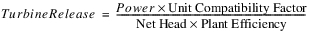7. The calculated Turbine Release is compared to the initial Turbine Release and the process iterates until the values converge.
Note:  Convergence Percentage is a general slot on power reservoirs representing the convergence in all iterative solutions; the slot defaults to 0.0001 if not user input.
Once converged, the Net Head is looked up on the Net Head Vs Max Turbine Release table to get the max release. If the Turbine Release is larger than the max release times the Power Plant Cap Fraction, the selected method in the Input Energy adjustment category is executed. The Reduce Input Energy method reduces the energy to the maximum possible. Otherwise, there is too much flow and an error will be issued that the energy request cannot be met.Peak Power Equation with Off Peak Spill Release
The Peak Power Equation with Off Peak Spill Release method calculates the necessary Turbine Release, Peak Release and Peak Time using the water power equation when Energy is specified. The method is only available if the Peak Power Equation with Off Peak Spill method is selected in the Power category. Energy must be input or set by a rule for this method to execute.
There are no slots specific to this method.
Method Details
This method first checks to see if Turbine Release is user input or set by a rule. If it is, the run terminates because both Energy and Turbine Release cannot be input. When the Energy value is known, the Peak Power Equation with Off Peak Spill Release method uses Energy to solve for Turbine Release, Peak Release and Peak Time as follows.
1. Peak Release is initially set to zero.
2. Given the net head from the previous timestep (Operating Head at previous timestep minus Head Loss), the efficiency is interpolated from the Net Head vs Efficiency table. The previous Operating Head is used as an approximation so as not to introduce an additional variable in the iteration. As a result, the Tailwater Elevation at the initial timestep must be input. The net head for the initial timestep is the initial Pool Elevation minus the initial Tailwater Elevation minus Head Loss.
3. The current Tailwater Elevation is determined using the maximum of Peak Release or the current Outflow as the value in the selected Tailwater method.
4. The Operating Head is calculated as the average Pool Elevation minus the Tailwater Elevation.
6. Given the net head, the Generator Capacity is interpolated from the Net Head vs. Generator Capacity table. If the capacity is above the Plant Power Limit, the Generator Capacity is reset to the Plant Power Limit.
7. Peak Release is calculated according to the power equation. The unit compatibility factor comes from balancing units and the specific weight of water; it is 102.01697767 in internal RiverWare units.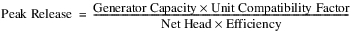8. The new Peak Release value is compared with the previous value and the iteration (steps 3-7) continues until the value converges.
Note:  Convergence Percentage is a general slot on power reservoirs representing the convergence in all iterative solutions; the slot defaults to 0.0001 if not input.
Power is set equal to the Generator Capacity and Peak Time is as follows: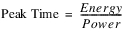Turbine Release is the Peak Release average over the timestep: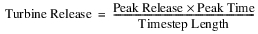Unit Generator Power Release
The Unit Generator Power Release method is only available when Unit Generator Power is selected in the Power category. It is used to calculate the Turbine Release required to produce a given amount of Power. Energy must be input by the user for this method to execute.
There are no slots specific to this method.
Method Details
If Energy is flagged UNIT VALUES (U), an error is issued. This flag is only available with the Unit Power Table Release method; see Unit Power Table Release.
The Unit Generator Power Release method begins by computing the availability and power limits of each unit type. Availability and power limit values are computed as the sum of the values from the availability and power limit columns, respectively, in the Generators Available and Limit slot. A value for availability and power limit is computed for each unit type.
The efficiency of each unit type is calculated by the following equation:PowerTemp and flowTemp, both local variables, are computed from the Best Generator Power and Best Generator Flow tables, respectively, using the current Operating Head. Each unit type is then sorted in descending order based on the computed efficiency.
In order to compute the Turbine Release associated with the known Power, the method begins to add entire unit types (operating according to the best power and flow tables and beginning with the most efficient type) until the Power is exceed or all the unit types have been added. If the Power is exceeded, the last generator type is interpolated to compute the Turbine Release exactly (see equation below). However, if all the unit types have been added and the Power cannot be met, the method assumes all unit types are operating at full capacity (according to the Full Generator Flow and Full Generator Power tables). Then if the Power is exceeded, the last generator type added is interpolated to compute the Power exactly (see equation below). However, if the Power still cannot be met, an error is posted and the run is terminated because the generators are unable to produce the amount of Power specified by the user.
The interpolation equation used to calculate Power is given below:where oneLessTypePower is the power produced from all the previous types added (excluding the most recent type added); oneLessTypeFlow is the flow through all the previous unit types (excluding the most recent type added); cumulativePower is the power produced from all the unit types added (including the most recent type); and cumulativeFlow is the flow through all the unit types added (including the most recent type).
Note:  This equation assumes the relationship between power and flow is linear regardless of the actual relationship specified in the power and flow tables. It is also interpolating over an entire type of generators.LCR Power Release
The LCR Power Release method calculates the release from the Lower Colorado River hydropower products. The LCR Power Release method is available only when LCR Power is selected in the Power category. Energy must be input or flagged as BEST EFFICIENCY (Energy cannot be flagged MAX CAPACITY for the LCR Power method) for this method to execute. It is determined if the requested Power demand can be met. This determination is based on the maximum possible power that can be generated for a given head. If it is possible to meet the requested Power demand, the Turbine Release is set so as to produce the requested Power.
If Energy is flagged UNIT VALUES (U), an error is issued. This flag is only available with the Unit Power Table Release method; see Unit Power Table Release.
There are no slots specific to this method.
Method Details
The first step in this method to verify the Lower Colo Power Coeffs are known. If either of these coefficients are not known, a RiverWare error is flagged and the simulation run is terminated. Then, the LCR Input Efficiency slot is checked. If it is not known, it is assumed to be 100% efficient and the LCR Input Efficiency is set to 1.0.
If Energy is flagged as BEST EFFICIENCY, it is calculated as the Net Energy Request plus the value of energy in the Station Energy Table corresponding to the current day of the week.
If Energy is flagged as MAX CAPACITY, an error is given. If Energy is not flagged as either BEST EFFICIENCY or MAX CAPACITY, it must be input by the user.
Turbine Release is calculated using the following equation:where the Timestep is in hours. The constants used in this equation are to convert the input to RiverWare standard units.
The previous equation is based on the energy calculation equation solved for Flow and corrected to standard units (see LCR Power method):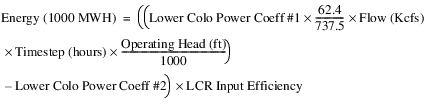where flow is in kcfs, Timestep is in hour, and Operating Head is in feet.
The correction factors used in these equations are presented below:Once Turbine Release is calculated, it is checked against the maximum allowable turbine release. A RiverWare error is flagged and the simulation run is terminated if Turbine Release exceeds the maximum allowable turbine release.Unit Power Table Release
This method is only available if the Unit Power Table method is selected in the Power category; see Unit Power Table for details. The method Unit Power Table Release calculates Turbine Release when Energy is specified. If Energy is flagged as BEST EFFICIENCY (B) or MAX CAPACITY (M) or UNIT VALUES (U), it is considered input.
If Energy is flagged B, the Unit Best Turbine Q table will be used to determine the best efficiency Turbine Release for the current average Operating Head. This assumes that all units are in use unless specified otherwise in the Unit is Generating slot. Power is then found using the Unit Power Table. If Energy is flagged M, the Unit Max Turbine Q table is used to determine the maximum Unit Turbine Release for the current average Operating Head. This point is then found in the Unit Power Table to determine the maximum power that can be produced for this Operating Head. If Energy is flagged U, the method calculates Unit Turbine Release using table interpolation of Unit Energy on the Unit Power Table with the Unit Energy.
If Energy is input but not flagged as B, M, or U and Unit Energy is not input, the method will exit without calculating Unit Energy. If any of the values in Unit Energy are input, it will be used to determine the release and power.
Method Details
This method will be called if Energy is input or set by a rule, which includes being flagged B, M, or U. This method will execute in the following manner.
if (Energy is flagged M)
{
If any of the Unit Energy[u] values are input or set by a rule, issue an error.
For each unit that is available (based on a non-zero value in the Unit is Generating slot), use 2D interpolation of Auto Unit Max Turbine Q table;
Set max release to a temporary local variable, Qmax[u];
Turbine Release is set to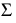Qmax[u];
Once the value for each unit flow at the current average Operating Head is found, the Unit Power[u] produced for that flow can be determined directly from the Unit Power Table.
}
else if (Energy is flagged B)
{
If any of the Unit Energy[u] values are input or set by a rule, issue an error.
For each unit that is available (based on a non-zero value in the Unit is Generating slot), use 2D interpolation of Auto Unit Best Turbine Q table to determine release at B;
Set best release to a temporary local variable, Qbest[u];
Turbine Release is set toQbest[u];
Again, Unit Power[u] will then be able to be determined directly from the Unit Power Table.
}
else if (Energy is Input/Rules (including “U” flag) and Unit Energy for any unit is not input/rules)
{
Issue an error; there is no way to calculate Unit Energy from plant values and no way to calculate plant Power without unit information
}
else if (Energy is input/rules (including “U” flag) and Unit Energy for any unit is input/rules)
{
If Unit is Generating is set (input/rules) to 0 for a unit that has a Unit Energy, issue an error.
If Unit is Generating is set (input/rules) to 1 for a unit that does not have a Unit Energy, issue an error.
If Energy is flagged U,; otherwise, if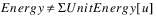, an error is issued
Next, Unit Power[u] = Unit Energy[u] / time (hrs)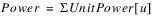From this power calculation, the Unit Turbine Release[u] can then be determined using a reverse table lookup of Unit Power[u] in the Unit Power Table. If the Shared Penstock Head Loss method is selected, the solution is iterative as the net operating head is a function of Turbine Release. If Unit Energy[u] is less than zero, the Unit Turbine Release[u] is set to zero. A negative Unit Energy can be set to represent a unit that is spinning but not producing power; that is condensing.
Turbine Release =Unit Turbine Release[u]
}
Finally, the method returns to the Unit Power Table method and computes Unit is Generating and Number of Units Generating. See Unit Power Table for details.
Revised: 08/02/2021